# 1.6: Determining Molecular Shape

$$\newcommand{\vecs}{\overset { \rightharpoonup} {\mathbf{#1}} }$$ $$\newcommand{\vecd}{\overset{-\!-\!\rightharpoonup}{\vphantom{a}\smash {#1}}}$$$$\newcommand{\id}{\mathrm{id}}$$ $$\newcommand{\Span}{\mathrm{span}}$$ $$\newcommand{\kernel}{\mathrm{null}\,}$$ $$\newcommand{\range}{\mathrm{range}\,}$$ $$\newcommand{\RealPart}{\mathrm{Re}}$$ $$\newcommand{\ImaginaryPart}{\mathrm{Im}}$$ $$\newcommand{\Argument}{\mathrm{Arg}}$$ $$\newcommand{\norm}{\| #1 \|}$$ $$\newcommand{\inner}{\langle #1, #2 \rangle}$$ $$\newcommand{\Span}{\mathrm{span}}$$ $$\newcommand{\id}{\mathrm{id}}$$ $$\newcommand{\Span}{\mathrm{span}}$$ $$\newcommand{\kernel}{\mathrm{null}\,}$$ $$\newcommand{\range}{\mathrm{range}\,}$$ $$\newcommand{\RealPart}{\mathrm{Re}}$$ $$\newcommand{\ImaginaryPart}{\mathrm{Im}}$$ $$\newcommand{\Argument}{\mathrm{Arg}}$$ $$\newcommand{\norm}{\| #1 \|}$$ $$\newcommand{\inner}{\langle #1, #2 \rangle}$$ $$\newcommand{\Span}{\mathrm{span}}$$

## Bond lengths and angles

The length of a chemical bond the distance between the centers of the two bonded atoms (the internuclear distance.) Bond lengths have traditionally been expressed in Ångstrom units, but picometers are now preferred (1Å = 10-8 cm = 100 pm.) Bond lengths are typically in the range 1-2 Å or 100-200 pm. Even though the bond is vibrating, equilibrium bond lengths can be determined experimentally to within ±1 pm.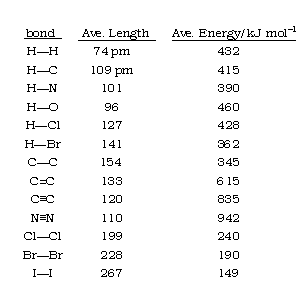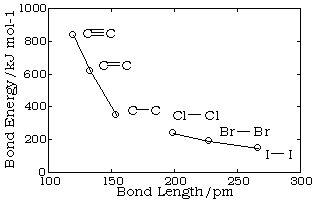Bond lengths depend mainly on the sizes of the atoms, and secondarily on the bond strengths, the stronger bonds tending to be shorter. Bonds involving hydrogen can be quite short; The shortest bond of all, H–H, is only 74 pm. Multiply-bonded atoms are closer together than singly-bonded ones; this is a major criterion for experimentally determining the multiplicity of a bond. This trend is clearly evident in the above plot which depicts the sequence of carbon-carbon single, double, and triple bonds.

The most common method of measuring bond lengths in solids is by analysis of the diffraction or scattering of X-rays when they pass through the regularly-spaced atoms in the crystal. For gaseous molecules, neutron- or electron-diffraction can also be used.The complete structure of a molecule requires a specification of the coordinates of each of its atoms in three-dimensional space. This data can then be used by computer programs to construct visualizations of the molecule as discussed above. One such visualization of the water molecule, with bond distances and the HOH bond angle superimposed on a space-filling model, is shown here. The colors show the results of calculations that depict the way in which electron charge is distributed around the three nuclei.

In most cases the focus of configuration is a carbon atom so the lines specifying bond directions will originate there. As defined in the diagram on the right, a simple straight line represents a bond lying approximately in the surface plane. The two bonds to substituents A in the structure on the left are of this kind. A wedge shaped bond is directed in front of this plane (thick end toward the viewer), as shown by the bond to substituent B; and a hatched bond is directed in back of the plane (away from the viewer), as shown by the bond to substituent D. Some texts and other sources may use a dashed bond in the same manner as we have defined the hatched bond, but this can be confusing because the dashed bond is often used to represent a partial bond (i.e. a covalent bond that is partially formed or partially broken).

## Molecular Shapes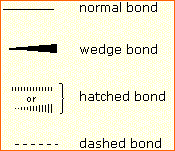The following examples make use of this notation, and also illustrate the importance of including non-bonding valence shell electron pairs (colored blue) when viewing such configurations.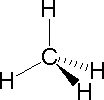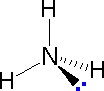Methane Ammonia Water

Bonding configurations are readily predicted by valence-shell electron-pair repulsion theory, commonly referred to as VSEPR in most introductory chemistry texts. This simple model is based on the fact that electrons repel each other, and that it is reasonable to expect that the bonds and non-bonding valence electron pairs associated with a given atom will prefer to be as far apart as possible. The bonding configurations of carbon are easy to remember, since there are only three categories.

Configuration Bonding Partners Bond Angles Example
Tetrahedral 4 109.5ºTrigonal 3 120º
Linear 2 180º

In the three examples shown above, the central atom (carbon) does not have any non-bonding valence electrons; consequently the configuration may be estimated from the number of bonding partners alone. For molecules of water and ammonia, however, the non-bonding electrons must be included in the calculation. In each case there are four regions of electron density associated with the valence shell so that a tetrahedral bond angle is expected. The measured bond angles of these compounds (H2O 104.5º & NH3 107.3º) show that they are closer to being tetrahedral than trigonal or linear. Of course, it is the configuration of atoms (not electrons) that defines the the shape of a molecule, and in this sense ammonia is said to be pyramidal (not tetrahedral). The compound boron trifluoride, BF3, does not have non-bonding valence electrons and the configuration of its atoms is trigonal. The best way to study the three-dimensional shapes of molecules is by using molecular models. Many kinds of model kits are available to students and professional chemists.

## Two Electron Groups

Our first example is a molecule with two bonded atoms and no lone pairs of electrons,BeH2

### AX2: BeH2

1. The central atom, beryllium, contributes two valence electrons, and each hydrogen atom contributes one. The Lewis electron structure is2. There are two electron groups around the central atom. We see from Figure 9.2.2 that the arrangement that minimizes repulsions places the groups 180° apart.

3. Both groups around the central atom are bonding pairs (BP). Thus BeH2 is designated as AX2.

4. From Figure 9.2.3 we see that with two bonding pairs, the molecular geometry that minimizes repulsions in BeH2 is linear.

### AX2: CO2

1. The central atom, carbon, contributes four valence electrons, and each oxygen atom contributes six. The Lewis electron structure is2. The carbon atom forms two double bonds. Each double bond is a group, so there are two electron groups around the central atom. Like BeH2, the arrangement that minimizes repulsions places the groups 180° apart.

3. Once again, both groups around the central atom are bonding pairs (BP), so CO2 is designated as AX2.

4. VSEPR only recognizes groups around the central atom. Thus the lone pairs on the oxygen atoms do not influence the molecular geometry. With two bonding pairs on the central atom and no lone pairs, the molecular geometry of CO2 is linear (Figure 9.2.3). The structure of CO2 is shown in Figure 9.2.2.1.

## Three Electron Groups

### AX3: BCl3

1. The central atom, boron, contributes three valence electrons, and each chlorine atom contributes seven valence electrons. The Lewis electron structure is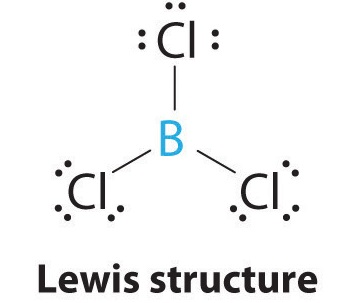2. There are three electron groups around the central atom. To minimize repulsions, the groups are placed 120° apart (Figure 9.2.2).

3. All electron groups are bonding pairs (BP), so the structure is designated as AX3.

4. From Figure 9.2.3 we see that with three bonding pairs around the central atom, the molecular geometry of BCl3 is trigonal planar, as shown in Figure 9.2.2.1.

### AX3: CO32−

1. The central atom, carbon, has four valence electrons, and each oxygen atom has six valence electrons. As you learned previously, the Lewis electron structure of one of three resonance forms is represented as2. The structure of CO32− is a resonance hybrid. It has three identical bonds, each with a bond order of 113. We minimize repulsions by placing the three groups 120° apart (Figure 9.2.2).

3. All electron groups are bonding pairs (BP). With three bonding groups around the central atom, the structure is designated as AX3.

4. We see from Figure 9.2.3 that the molecular geometry of CO32− is trigonal planar.In our next example we encounter the effects of lone pairs and multiple bonds on molecular geometry for the first time.

### AX2E: SO2

1. The central atom, sulfur, has 6 valence electrons, as does each oxygen atom. With 18 valence electrons, the Lewis electron structure is shown below.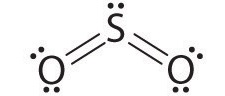2. There are three electron groups around the central atom, two double bonds and one lone pair. We initially place the groups in a trigonal planar arrangement to minimize repulsions (Figure 9.2.2).

3. There are two bonding pairs and one lone pair, so the structure is designated as AX2E. This designation has a total of three electron pairs, two X and one E. Because a lone pair is not shared by two nuclei, it occupies more space near the central atom than a bonding pair (Figure 9.2.4). Thus bonding pairs and lone pairs repel each other electrostatically in the order BP–BP < LP–BP < LP–LP. In SO2, we have one BP–BP interaction and two LP–BP interactions.

4. The molecular geometry is described only by the positions of the nuclei, not by the positions of the lone pairs. Thus with two nuclei and one lone pair the shape is bent, or V shaped, which can be viewed as a trigonal planar arrangement with a missing vertex (Figures 9.2.2.1 and 9.2.3).Figure 9.2.4: The Difference in the Space Occupied by a Lone Pair of Electrons and by a Bonding Pair

As with SO2, this composite model of electron distribution and negative electrostatic potential in ammonia shows that a lone pair of electrons occupies a larger region of space around the nitrogen atom than does a bonding pair of electrons that is shared with a hydrogen atom.

Like lone pairs of electrons, multiple bonds occupy more space around the central atom than a single bond, which can cause other bond angles to be somewhat smaller than expected. This is because a multiple bond has a higher electron density than a single bond, so its electrons occupy more space than those of a single bond. For example, in a molecule such as CH2O (AX3), whose structure is shown below, the double bond repels the single bonds more strongly than the single bonds repel each other. This causes a deviation from ideal geometry (an H–C–H bond angle of 116.5° rather than 120°).## Four Electron Groups

One of the limitations of Lewis structures is that they depict molecules and ions in only two dimensions. With four electron groups, we must learn to show molecules and ions in three dimensions.

### AX4: CH4

1. The central atom, carbon, contributes four valence electrons, and each hydrogen atom has one valence electron, so the full Lewis electron structure is2. There are four electron groups around the central atom. As shown in Figure 9.2.2, repulsions are minimized by placing the groups in the corners of a tetrahedron with bond angles of 109.5°.

3. All electron groups are bonding pairs, so the structure is designated as AX4.

4. With four bonding pairs, the molecular geometry of methane is tetrahedral (Figure 9.2.3).### AX3E: NH3

1. In ammonia, the central atom, nitrogen, has five valence electrons and each hydrogen donates one valence electron, producing the Lewis electron structure2. There are four electron groups around nitrogen, three bonding pairs and one lone pair. Repulsions are minimized by directing each hydrogen atom and the lone pair to the corners of a tetrahedron.

3. With three bonding pairs and one lone pair, the structure is designated as AX3E. This designation has a total of four electron pairs, three X and one E. We expect the LP–BP interactions to cause the bonding pair angles to deviate significantly from the angles of a perfect tetrahedron.

4. There are three nuclei and one lone pair, so the molecular geometry is trigonal pyramidal. In essence, this is a tetrahedron with a vertex missing (Figure 9.2.3). However, the H–N–H bond angles are less than the ideal angle of 109.5° because of LP–BP repulsions (Figure 9.2.3 and Figure 9.2.4).

### AX2E2: H2O

1. Oxygen has six valence electrons and each hydrogen has one valence electron, producing the Lewis electron structure2. There are four groups around the central oxygen atom, two bonding pairs and two lone pairs. Repulsions are minimized by directing the bonding pairs and the lone pairs to the corners of a tetrahedron Figure 9.2.2.

3. With two bonding pairs and two lone pairs, the structure is designated as AX2E2 with a total of four electron pairs. Due to LP–LP, LP–BP, and BP–BP interactions, we expect a significant deviation from idealized tetrahedral angles.

4. With two hydrogen atoms and two lone pairs of electrons, the structure has significant lone pair interactions. There are two nuclei about the central atom, so the molecular shape is bent, or V shaped, with an H–O–H angle that is even less than the H–N–H angles in NH3, as we would expect because of the presence of two lone pairs of electrons on the central atom rather than one.. This molecular shape is essentially a tetrahedron with two missing vertices.## ContributorsThis page titled 1.6: Determining Molecular Shape is shared under a not declared license and was authored, remixed, and/or curated by Layne Morsch.